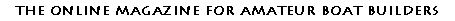NEW HOME INPUT INDEXES FEATURES CLASSIFIED CONTESTS ARCHIVES FORUM STORE LINKS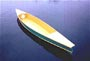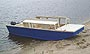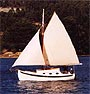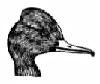Bird’s Mouth Spars The Real Equations By David FarlessIntroduction I recently became interested in the Bird’s Mouth technique for hollow spar construction after encountering web sites where the technique was being applied. One of these is a site called Building of Aslan (author/owner Frank Hagan) and the other is an article in DuckWorks Magazine (A Birdsmouth Mast for Skat by Dave Burdecki). The Bird’s Mouth technique for hollow spar construction uses 8 wooden staves of rectangular cross section, each with a 90º notch cut in one edge. The staves are glued together by nesting a corner of one stave into the notch of the next stave. The Aslan web site presents basic “rule-of-thumb” methods for calculating the dimensions of the staves. The stave cross sectional dimensions are arrived at by first dividing the desired spar outside diameter (O.D.) by 2.5 to get the width of the stave cross section and then setting the stave depth at 1/2 of the width. The Aslan article cites WoodenBoat Magazine (WoodenBoat, Number 149, July/August, 1999) as the source of the rules-of-thumb. I decided to order the back issue of WoodenBoat to see the mathematics behind these basic calculations. The article in WoodenBoat is “Bird’s Mouth Hollow Spars”, by Aime´ Ontario Fraser. Unfortunately, the only math presented is in a side-bar titled Scantlings for Bird’s Mouth Spars which contains two formulas. These formulas are: stave width = 0.4 x O.D. stave depth = 0.2 x O.D. As expected, these formulas match the rules-of-thumb used in the Aslan web site, since multiplying by 0.4 is the same as dividing by 2.5 and 0.2 is half of 0.4. These rules-of-thumb for Bird’s Mouth spar development seem to work, but are not supported by any hard analysis. The WoodenBoat article ascribes them to a mysterious “retired engineer” who passed them to two Connecticut boat builders (Craig Wright and Richard Duke) and then disappeared. Being a retired engineer myself, I decided that I should be able to repeat the analysis by which these rules-of-thumb were derived. What follows here is that derivation. The Geometry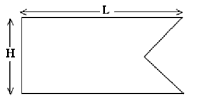The cross-sectional shape of the staves for the Bird’s Mouth spar is shown in this figure. The shape is a rectangle of width L and depth H, with a 90º notch cut out of one edge. The technique under discussion here uses 8 of these staves to make an approximately circular spar section. (More or fewer staves may be used, but this analysis only considers the case of 8 staves.) A cross section of the resulting spar is shown below. (Only 3 of the 8 staves are shown, for simplicity.) In this figure, R is the radius corresponding to the outer diameter of the spar (O.D.), R1 is the distance from the center of the spar to the inner edge of the staves, and R2 in the radius corresponding to the inner diameter of the spar (I.D.). Defining R2 as the inner radius of the spar rather than R1 is a conservative assumption (from the standpoint of strength) in that it ignores the additional wood left inside of this circle (unless the spar is reamed out to a circular inside diameter).The angle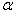in this figure is just the full circle divided by the number of staves; i.e.The Equations Based on the desired O.D. and I.D. of the spar, the dimensions of the stave cross section may be calculated from the following equations: Given O.D. and I.D., calculate L and H,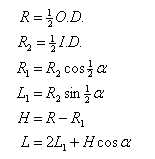Alternatively, if slats of a given cross section are to be used, the O.D. and I.D. of the resulting spar can be calculated as follows: Given L and H, calculate O.D. and I.D.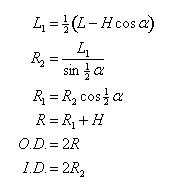Additional parameters of interest.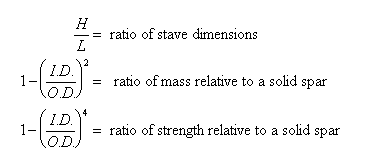Mass is proportional to the cross-sectional area of the spar. Strength is proportional to the moment of inertia of the cross-section of the spar. For a clear, entry-level discussion of relative mass and strength for hollow spars, see Jim Michalak’s discussion of hollow spars in his newsletter from February 2000.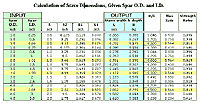The Calculated Results

The table at right (click to enlarge) shows the results of these calculations for spars with O.D. of 1, 2, 3, and 4inches and with varying I.D. values.

After using the WoodenBoat article rules-of-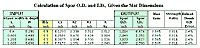thumb to calculate stave dimensions for spars with O.D. of 1, 2, 3, and 4 inches, the second set of formulas was used to calculate the actual resulting spar diameters. The next table shows the results. (click to enlarge)

The Analysis

Note in the first table that for all spar O.D. values, if the ratio H/L is 0.500 (see entries highlighted in yellow) then the mass relative to a solid spar is always 56 % and the strength is always 81% of that for a solid spar. This is also shown in the second table where L and H have been selected in all four calculations by using the rules-of thumb as given in WoodenBoat. The rules-of-thumb are said to provide stave dimensions giving spars with an O.D. of 1, 2, 3, and 4 inches. However, the actual spar O.D. is always 2.4% high or about 1/16 inch for a 3-inch spar. This error of less than 1/16 inch for spars of less than 3-inch O.D. is well within acceptable error for small boat builders.

I have not tried to verify the wall thickness needed to meet accepted scantlings for wooden boat spars. However, the WoodenBoat article quotes the Connecticut boat builders (Wright and Duke) as saying that “the formula to determine the thickness of the staves perfectly matches the recommendations for hollow round masts in Skene’s Elements of Yacht Design by Francis S. Kinney.” The Connecticut builders are further quoted in the WoodenBoat article, as follows:

 “Wright and Duke feel that this formula results in an excessive wall thickness for small-craft masts and is more appropriate for larger yachts having spars of spruce. Their experience has proven that you can make the thickness as little as 0.10 times the major diameter for very small, light boats. They use a factor of 0.15 for clear Douglas fir, since it is stronger than spruce in every way.”

So, it seems safe, and perhaps overly conservative, to apply these rules-of-thumb for development of hollow wooden spars for small boats.

The Summary

This analysis supports the use of the mysterious engineer’s rules-of-thumb for Bird’s Mouth spar development as presented in WoodenBoat. He had obviously “done his work” well as spars resulting from the use of his rules-of-thumb will result in a circular spar very near the intended dimensions and with more than adequate strength for small boats. And they will give a reduction in spar weight of about 44% while retaining 81% of the strength of a solid spar. (A 62% improvement in the strength-to-weight ratio!)

Given that the “Rules-of-Thumb” can provide reasonable and useable stave dimensions, the equations presented here may seem to be over-kill and unnecessary. However, they can be useful in at least two situations. First, they can be used to determine how much to taper the staves (reduction in L) to achieve a given O.D. at the end of a tapered spar. Second, they can be used to calculate the inside diameter resulting from given stave dimensions, allowing estimates to be made of the strength and mass of the spar.

And, perhaps most importantly, they provide a documented basis to support knowledgeable use of the rules-of-thumb.